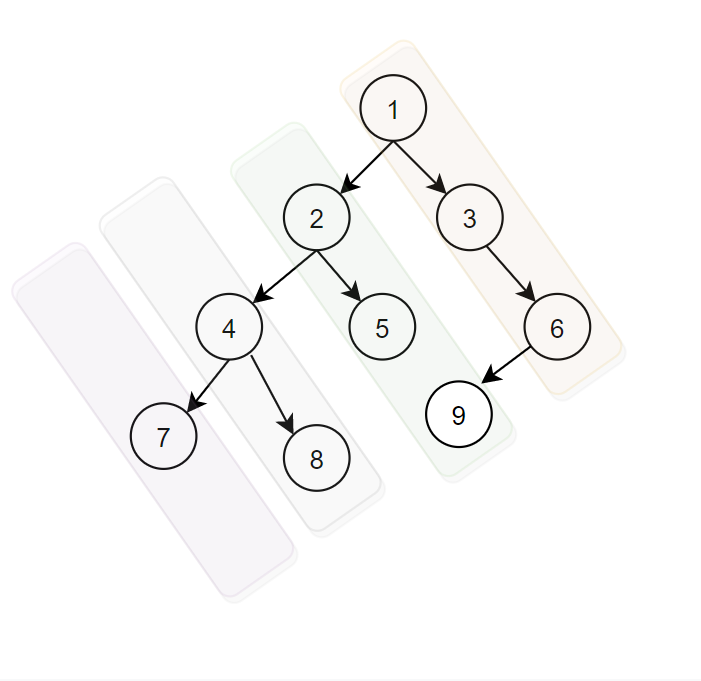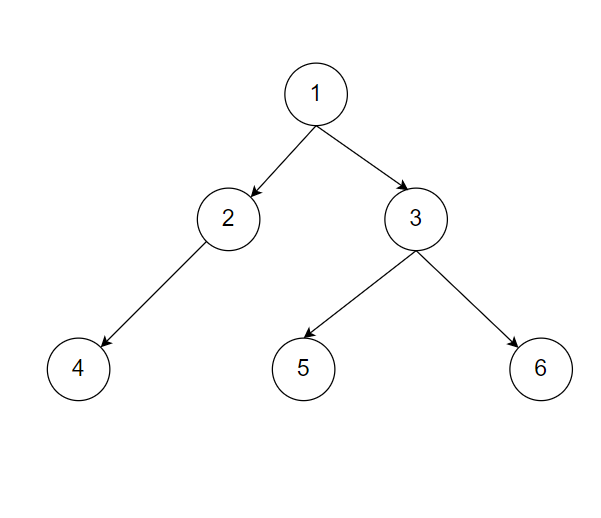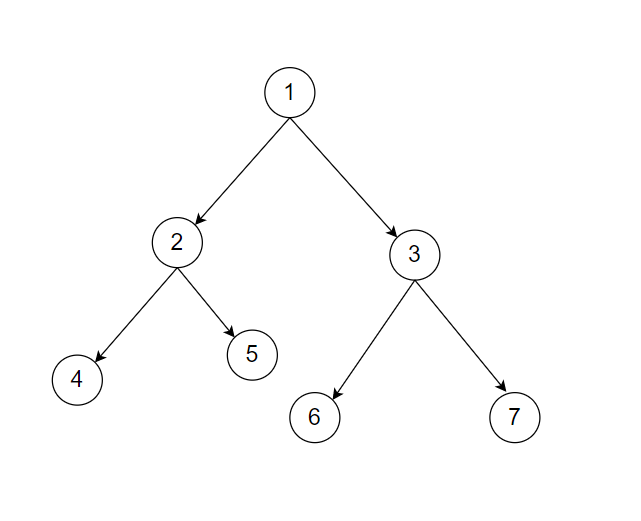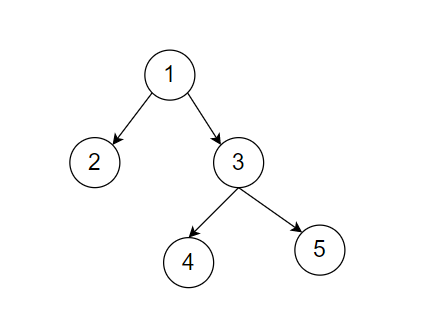1

# Diagonal Traversal

Difficulty: EASY
Avg. time to solve
20 min
Success Rate
80%

Problem Statement
Suggest Edit

#### Consider this binary tree``````There are 4 diagonal path
1 3 6
2 5 9
4 8
7
``````
##### Note:
``````Do not print anything, just return an array/vector of all diagonal paths.

Let's consider this example
````````````diagonal path is
1 3 6
2 5
4

Then return ‘1 3 6 2 5 4’

Order of return diagonal path’s array/vector, the rightmost diagonal path must come first, and so on.

Every parent node comes first then the child node in other words prints the diagonal element from top to bottom.
``````
##### Input Format:
``````The first line of input contains an integer ‘T’ denoting the number of test cases.
The next ‘T’ lines represent the ‘T’ test cases.

The first line of input contains the elements of the tree in the level order form separated by a single space.
If any node does not have a left or right child, take -1 in its place. Refer to the example below.

Example:

Elements are in the level order form. The input consists of values of nodes separated by a single space in a single line. In case a node is null, we take -1 in its place.

For example, the input for the tree depicted in the below image would be :
```````````` 1
2 3
4 -1 5 6
-1 7 -1 -1 -1 -1
-1 -1

Explanation :
Level 1 :
The root node of the tree is 1

Level 2 :
Left child of 1 = 2
Right child of 1 = 3

Level 3 :
Left child of 2 = 4
Right child of 2 = null (-1)
Left child of 3 = 5
Right child of 3 = 6

Level 4 :
Left child of 4 = null (-1)
Right child of 4 = 7
Left child of 5 = null (-1)
Right child of 5 = null (-1)
Left child of 6 = null (-1)
Right child of 6 = null (-1)

Level 5 :
Left child of 7 = null (-1)
Right child of 7 = null (-1)

The first not-null node (of the previous level) is treated as the parent of the first two nodes of the current level. The second not-null node (of the previous level) is treated as the parent node for the next two nodes of the current level and so on.

The input ends when all nodes at the last level are null (-1).
``````
##### Note :
``````The above format was just to provide clarity on how the input is formed for a given tree.

The sequence will be put together in a single line separated by a single space. Hence, for the above-depicted tree, the input will be given as:

1 2 3 4 -1 5 6 -1 7 -1 -1 -1 -1 -1 -1
``````
##### Output Format:
``````For every test case, return an array/vector containing diagonal paths in which the rightmost diagonal path comes first, the second rightmost diagonal path comes second, and so on.
``````
##### Constraint :
``````1 <= T <= 100
1 <= N <= 3000
-10^9 <= data <= 10^9

Where ‘T’ represents the number of test cases, ‘N’ is the number of nodes in the tree, and data denotes data contained in the node of a binary tree.

Time Limit: 1 sec
``````
##### Sample Input 1:
``````2
1 2 3 4 5 6 7 -1 -1 -1 -1 -1 -1 -1 -1
1 2 3 -1 -1 4 5 -1 -1 -1 -1
``````
##### Sample output 1:
`````` 1 3 7 2 5 6 4
1 3 5 2 4
``````
##### Explanation to
``````Test case 1:
````````````It is clear that 1 3 7 are the first diagonal path 2 5 6 are the second and 4 is the third diagonal path. But 5 6 has the same level and same diagonal path, so we need to consider a node that comes first in the pre-order traversal.
Hence the diagonal path of the above binary tree is 1 3 7 2 5 6 4

Test case 2:
````````````It is a binary tree representation of ‘1 2 3 -1 -1 5 4 -1 -1 -1 -1’. So it is clear that the first diagonal path is 1 3 5, and the second is 2 4.
Hence diagonal binary tree traversal when combined is 1 3 5 2 4
``````
##### Sample Input 2:
``````2
1 -1 2 3 4 -1 -1 -1 -1
1 2 3 -1 -1 4 -1 -1 -1
``````
##### Sample output 2:
``````1 2 4 3
1 3 2 4
``````Want to solve this problem? Login now to get access to solve the problems